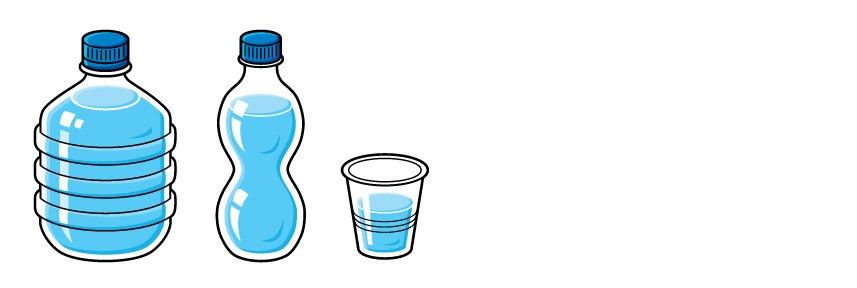How do we measure liquids?

When we measure liquids, we usually measure in millilitres (ml) and litres (L).

Have a look at this picture:The big bottle holds 1000 ml (1 L).

The small bottle holds 500 ml.

The cup holds 100 ml.

The big bottle can hold the most water and the cup can hold the least water.

1 litre (L) is the same as 1000 millilitres (ml).

2 L = 2000 ml

2.5 L = 2500 ml

An easy way to convert from litres to millilitres is to multiply the number by 1000.

4 L to ml: 4 L x 1000 = 4000 ml

To convert from millilitres to litres we divide the number by 1000.

5000 ml to L: 5000 ml ÷ 1000 = 5 L

Example 1

Convert 5 L to ml